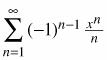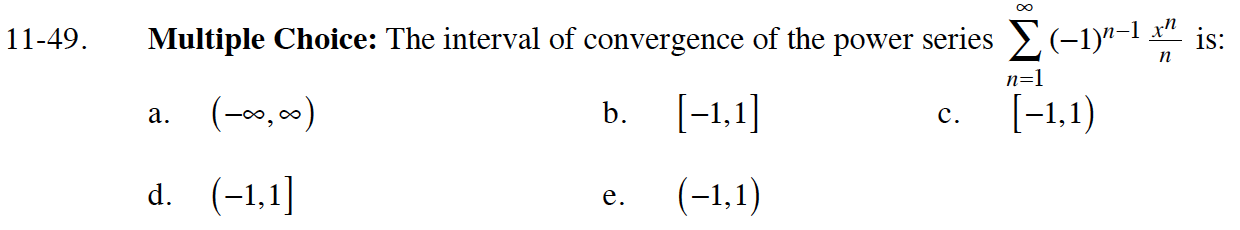### Home > CALC > Chapter 11 > Lesson 11.2.1 > Problem11-49

11-49.
1. Multiple Choice: The interval of convergence of the power seriesis: Homework Help ✎

1. (−∞, ∞)

2. [−1,1]

3. [−1,1)

4. (−1,1]

5. (−1,1)Use the Ratio Test.

$\lim_{n\to\infty}|x|\cdot\frac{n}{n+1}=$

If x = –1:

$\sum_{n=1}^{\infty}(-1)^{n-1}\frac{(-1)^n}{n}=\sum_{n=1}^{\infty}\frac{(-1)^{2n-1}}{n}=\sum_{n=1}^{\infty}(-1)^{n-1}\frac{(-1)}{n}$

Does this resultant series converge or diverge?
Be sure to check the resultant series if x = 1.